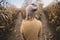# Overcoming Complexity With Agent Based Models

A beginners guide to agent based models: How they work and what they do

It is a sunny autumn afternoon.

You sit on a bench enjoying the last warm sun rays of the day.

In the distance you can see a large flock of birds. They are performing an artistic dance, drawing impressive figures in the air.

You may start to wonder: How does this work? The movements of the whole flock are so complex, how are they emerging from the behavior of each individual?

In our daily life we are surrounded by complex systems. A complex system is a…

# A Guide to the Regression of Rates and Proportions

## Modelling probabilities using beta regression in Python

Often in real world regression problems the target variable may be a probability or a proportion. You can think of modelling read ratios of your articles, the win-ratio of your favorite soccer team or customer churn rates of your enterprise. All of these problems have in common that the target variable is bound between 0 and 1.

In this post we will explore what kind of difficulties may arise in such a setting, why a standard linear regression may not be applicable and how to solve the problem.

# The Problem

Let's consider you want to model the click-through rate of an advertisement…

# Modelling a Pandemic

## Building an agent-based model using Python and pandas

In our daily life, we encounter many complex systems where individuals are interacting with each other such as the stock market or rush hour traffic. Finding appropriate models for these complex systems may give us a better understanding of their dynamics and allows us to simulate its behaviour under changing conditions. One way of modelling complex systems is by using agent-based models, meaning that we are explicitly simulating individuals and their interactions instead of deriving the dynamics of the system in an aggregate way.

You can find an introductory article about agent based models in general here:

In this post…

# Introduction

Bayesian analysis offers the possibility to get more insights from your data compared to the pure frequentist approach. In this post, I will walk you through a real life example of how a Bayesian analysis can be performed. I will demonstrate what may go wrong when choosing a wrong prior and we will see how we can summarize our results. For you to follow this post, I assume you are familiar with the foundations of Bayesian statistics and with Bayes' theorem.

## Scenario

As an example analysis, we will discuss a real life problem from a physics lab. No worries, you don't…

# How to Become a Bayesian

## On the fundamental difference between frequentist and Bayesian statisticsThe difference between the Bayesian and the frequentist approach lies in the interpretation of probability. Photo by Burst on Unsplash

The Bayesian approach to statistics is a powerful alternative to the frequentist approach. In this post, we will explore the very foundations of the Bayesian viewpoint and how it can be distinguished from the frequentist viewpoint. We will not derive Bayes' theorem in a purely technical way, but try to understand the underlying principles.

It all starts with probability. Probability theory is the foundation of statistics and data analysis. It is already the definition, or the viewpoint, on probability where the way splits between the Bayesian and the frequentist approach. How can there be different viewpoints on probability, you may…## Christian Graf

Data Scientist | Particle Physicist